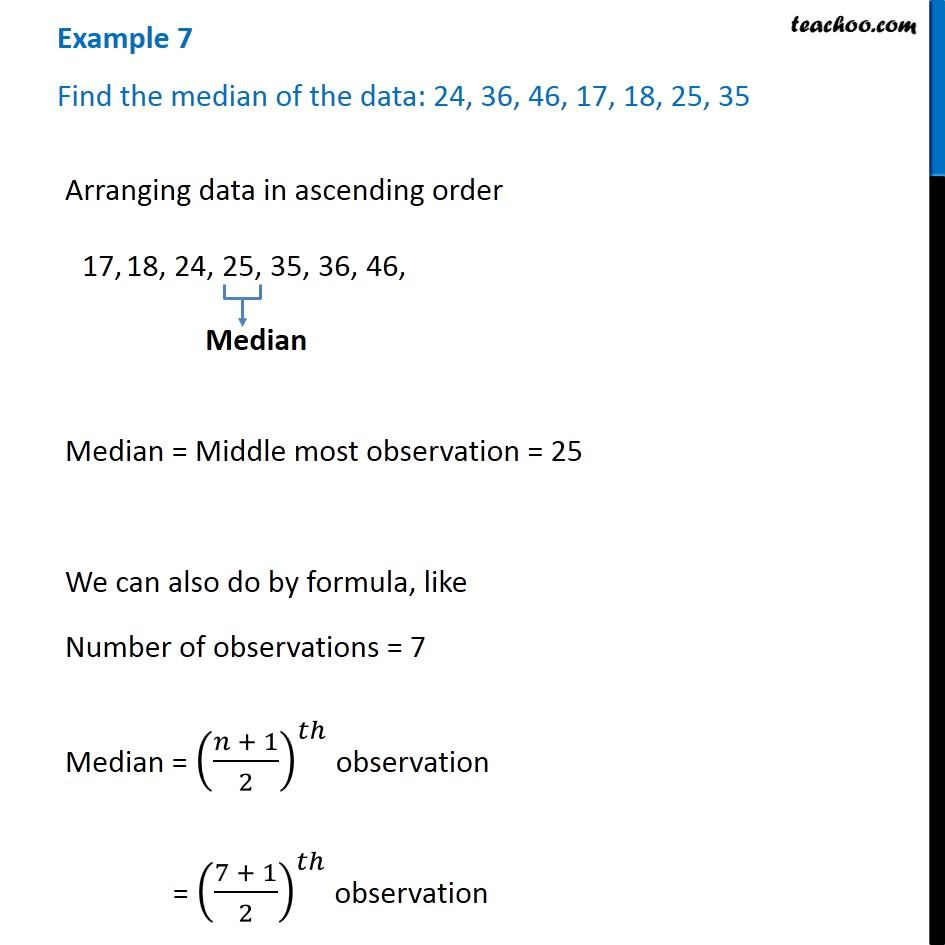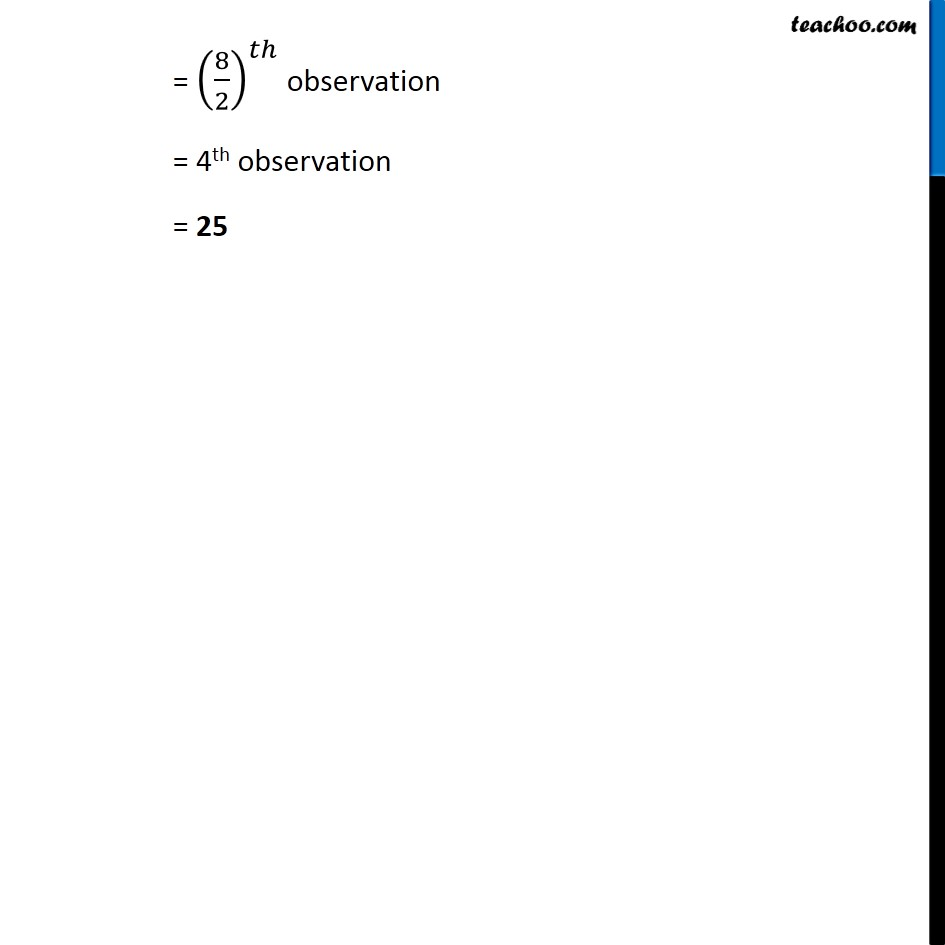Examples

Chapter 3 Class 7 Data Handling
Serial order wiseLearn in your speed, with individual attention - Teachoo Maths 1-on-1 Class

### Transcript

Example 7 Find the median of the data: 24, 36, 46, 17, 18, 25, 35 Arranging data in ascending order Median = Middle most observation = 25 We can also do by formula, like Number of observations = 7 Median = ((𝑛 + 1)/2)^𝑡ℎ observation = ((7 + 1)/2)^𝑡ℎ observation = (8/2)^𝑡ℎ observation = 4th observation = 25